https://blog.laffitto.xyz 乱谈府 ——詹詹碎言 2023-05-22T04:24:06.643Z https://github.com/jpmonette/feed Aspire to inspire until I expire 🎸 也扯淡，也思考，也生活 https://blog.laffitto.xyz/images/avatar.png https://blog.laffitto.xyz/favicon.ico All rights reserved 2023, 乱谈府 ——詹詹碎言 <![CDATA[八三夭live house]]> https://blog.laffitto.xyz/post/ba-san-yao-live-house/ 2023-05-22T02:17:36.000Z 八三夭的神奇体验

]]>

live house（ライブハウス）诞生于上世纪的日本，作为一种于体育馆截然不同的形式，live house为那些名气不是特别大的音乐人提供了一个很好的舞台，三五百人的容纳提供了更好的氛围与形式。这次去的奕空间正好位于合肥奥体中心旁边，是一个篮球场改造的，场馆内部是一整个空间，大概容纳了五百人左右。舞台空间上，音响质量还可以，正前方，左前方和右前方各有一个大屏幕，基本上就是体育馆演唱会的微缩版。

]]>
<![CDATA[OpenCV高阶操作]]> https://blog.laffitto.xyz/post/opencv-gao-jie-cao-zuo/ 2023-04-10T04:20:59.000Z 一些OpenCV的高级操作和代码

]]>

## 阈值处理

• 手动提供参数来设定合适的阈值分割图像。需要控制照明条件下工作得非常好，可以确保图像的前景和背景之间的高对比度。
• 如OTSU阈值之类的方法，这些方法试图更动态地自动计算最佳阈值。
• 自适应阈值，它不是试图用一个单一的值全局地阈值一个图像，而是把图像分解成更小的部分，然后分别地和单独地对每个部分进行阈值。

### 手动阈值 Basic thresholding

# apply basic thresholding -- the first parameter is the image
# we want to threshold, the second value is is our threshold
# check; if a pixel value is greater than our threshold (in this
# case, 200), we set it to be *black, otherwise it is *white*
(T, threshInv) = cv2.threshold(blurred, 200, 255,
cv2.THRESH_BINARY_INV)
cv2.imshow("Threshold Binary Inverse", threshInv)


cv2.threshold 第一个参数为需要处理的图像，第二个为阈值T，第三个为高于阈值后需要变化为的数值（通常为255，表示变为黑色），第四个为阈值处理方法。

### 自动化阈值 OTSU

OTUS可以帮助我们自动设置阈值。Otsu的方法假设我们的图像像素强度的灰度直方图是双模态的，这仅仅意味着直方图是两个峰。[[opencv直方图对比|直方图详解]]

(T, threshInv) = cv2.threshold(blurred, 0, 255,
cv2.THRESH_BINARY_INV | cv2.THRESH_OTSU)


import argparse
import cv2
ap = argparse.ArgumentParser()
help="path to input image")
args = vars(ap.parse_args())

# load the image and display it
cv2.imshow("Image", image)
# convert the image to grayscale and blur it slightly
gray = cv2.cvtColor(image, cv2.COLOR_BGR2GRAY)
blurred = cv2.GaussianBlur(gray, (7, 7), 0)

# apply Otsu's automatic thresholding which automatically determines
# the best threshold value
(T, threshInv) = cv2.threshold(blurred, 0, 255,
cv2.THRESH_BINARY_INV | cv2.THRESH_OTSU)
cv2.imshow("Threshold", threshInv)
print("[INFO] otsu's thresholding value: {}".format(T))
# visualize only the masked regions in the image
cv2.waitKey(0)


• cv2.THRESH_BINARY_INV 二值化后再反转
• cv2.THRESH_BINARY 正常二值化

thresh = cv2.adaptiveThreshold(blurred, 255,


image = cv2.imread('test.png')
cv2.imshow("Image", image)
# convert the image to grayscale and blur it slightly
gray = cv2.cvtColor(image, cv2.COLOR_BGR2GRAY)
blurred = cv2.GaussianBlur(gray, (7, 7), 0)

# instead of manually specifying the threshold value, we can use
# adaptive thresholding to examine neighborhoods of pixels and
cv2.waitKey(0)


## 图像平滑和模糊

### 平均模糊 cv2.blur

kernelSizes = [(3, 3), (9, 9), (15, 15)]
# loop over the kernel sizes
for (kX, kY) in kernelSizes:
# apply an "average" blur to the image using the current kernel
# size
blurred = cv2.blur(image, (kX, kY))
cv2.imshow("Average ({}, {})".format(kX, kY), blurred)
cv2.waitKey(0)


### 高斯模糊 cv2.GaussianBlur

x和y方向上的高斯函数如下：

$G(x,y)= \frac{1}{2 \pi \sigma}e^{- \frac{x^{2}+y^{2}}{2 \sigma ^{2}}}$

kernelSizes = [(3, 3), (9, 9), (15, 15)]
# loop over the kernel sizes again
for (kX, kY) in kernelSizes:
# apply a "Gaussian" blur to the image
blurred = cv2.GaussianBlur(image, (kX, kY), 0)
cv2.imshow("Gaussian ({}, {})".format(kX, kY), blurred)
cv2.waitKey(0)


### 中值模糊 cv2.medianBlur

kernelSizes = [(3, 3), (9, 9), (15, 15)]
# loop over the kernel sizes a final time
for k in (3, 9, 15):
# apply a "median" blur to the image
blurred = cv2.medianBlur(image, k)
cv2.imshow("Median {}".format(k), blurred)
cv2.waitKey(0)


### 双边模糊 cv2.bilateralFilter

blurred = cv2.bilateralFilter(image, diameter, sigmaColor, sigmaSpace)


## 形态学操作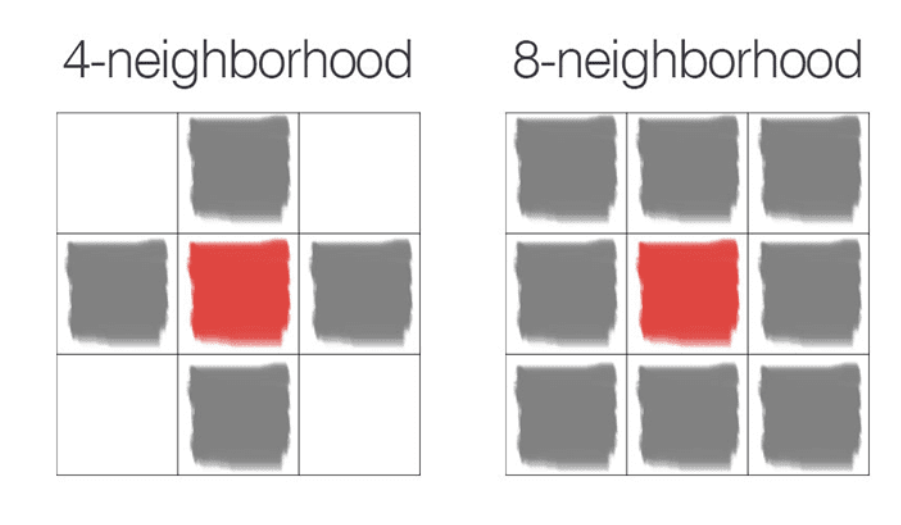### 侵蚀 Erosion

# apply a series of erosions
for i in range(0, 3):
eroded = cv2.erode(gray, None, iterations=i + 1)
cv2.imshow("Eroded {} times".format(i + 1), eroded)
cv2.waitKey(0)


### 膨胀 dilation

# apply a series of dilations
for i in range(0, 3):
dilated = cv2.dilate(gray.copy(), None, iterations=i + 1)
cv2.imshow("Dilated {} times".format(i + 1), dilated)
cv2.waitKey(0)



### 开操作 Opening

kernelSizes = [(3, 3), (5, 5), (7, 7)]
# loop over the kernels sizes
for kernelSize in kernelSizes:
# construct a rectangular kernel from the current size and then
# apply an "opening" operation
kernel = cv2.getStructuringElement(cv2.MORPH_RECT, kernelSize)
opening = cv2.morphologyEx(gray, cv2.MORPH_OPEN, kernel)
cv2.imshow("Opening: ({}, {})".format(
kernelSize, kernelSize), opening)
cv2.waitKey(0)


cv2.getStructuringElement用来定义一个结构元素。第一个参数结构元素类型：cv2.MORPH_RECT 表示正方形结构（8邻域），cv2.MORPH_CROSS 表示十字形结构（4邻域），cv2.MORPH_ELLIPSE 表示圆形结构。第二个参数表示大小。

### 闭操作 Closing

kernelSizes = [(3, 3), (5, 5), (7, 7)]
for kernelSize in kernelSizes:
# construct a rectangular kernel form the current size, but this
# time apply a "closing" operation
kernel = cv2.getStructuringElement(cv2.MORPH_RECT, kernelSize)
closing = cv2.morphologyEx(gray, cv2.MORPH_CLOSE, kernel)
cv2.imshow("Closing: ({}, {})".format(
kernelSize, kernelSize), closing)
cv2.waitKey(0)


kernelSizes = [(3, 3), (5, 5), (7, 7)]
for kernelSize in kernelSizes:
# construct a rectangular kernel and apply a "morphological
# gradient" operation to the image
kernel = cv2.getStructuringElement(cv2.MORPH_RECT, kernelSize)
cv2.waitKey(0)


cv2.morphologyEx 函数中方法选择 cv2.MORPH_GRADIENT

### 顶帽操作（Top hat/white hat）和黑帽操作（black hat）

top hat用于在深色背景上显示图像的明亮区域。

image = cv2.imread(args["image"])
gray = cv2.cvtColor(image, cv2.COLOR_BGR2GRAY)

# construct a rectangular kernel (13x5) and apply a blackhat
# operation which enables us to find dark regions on a light
# background
rectKernel = cv2.getStructuringElement(cv2.MORPH_RECT, (13, 5))
blackhat = cv2.morphologyEx(gray, cv2.MORPH_BLACKHAT, rectKernel)

# similarly, a tophat (also called a "whitehat") operation will
# enable us to find light regions on a dark background
tophat = cv2.morphologyEx(gray, cv2.MORPH_TOPHAT, rectKernel)

# show the output images
cv2.imshow("Original", image)
cv2.imshow("Blackhat", blackhat)
cv2.imshow("Tophat", tophat)
cv2.waitKey(0)


## 颜色空间

### 照明条件的重要性

• 高对比度 High contrast
尽量确保环境的背景和前景之间有很高的对比度，这将使编写代码更容易准确地理解背景和前景。

• 可推广的 Generalizable
照明条件应该足够一致，以至于它们从一个物体到另一个对象都可以很好地工作。

• 稳健性 Stable
拥有稳定、一致和可重复的照明条件是计算机视觉应用开发重要因素。

### RGB color space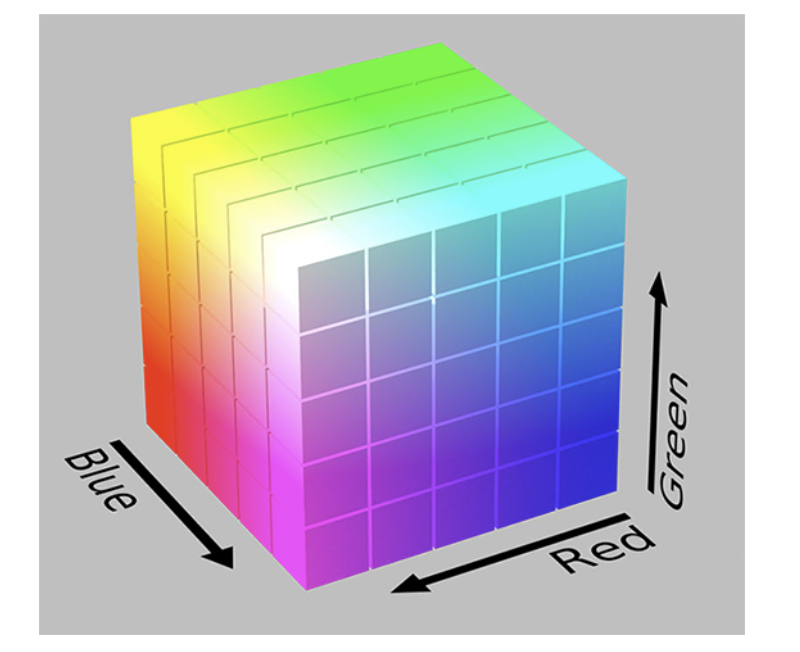### HSV color space

HSV颜色空间会改变RGB的颜色空间，将其重塑为圆柱体而不是立方体。在RGB部分中看到的，颜色的白色或明度是每个红色、绿色和蓝色组件的相加组合。但现在在HSV颜色空间中，亮度被赋予了自己的独立维度。

• Hue：检查哪种“纯”颜色。例如，颜色“红色”的所有阴影和色调都具有相同的色调。

• Saturation：颜色是多么“白色”。完全饱和的颜色将是“纯”，如“纯红色”。零饱和的颜色将是纯白色。

• value：控制颜色的明度。0将表示纯黑色，而增加将产生较浅的颜色

hsv = cv2.cvtColor(image, cv2.COLOR_BGR2HSV)


Value组件本质上是一个灰度图像，这是因为Value控制了颜色的实际明度，而Hue和Saturation定义了实际的颜色和阴影。HSV颜色空间在计算机视觉应用中被大量使用，特别是当我们对跟踪图像中某些物体的颜色感兴趣时。使用HSV定义一个有效的颜色范围比使用RGB要容易得多。

### L* a* b* color space

• L-channel: 像素的亮度。这个值在垂直轴上上下变化，从白色到黑色，轴的中心是中性灰色。
• a-channel: 源于L通道的中心，在光谱的一端定义纯绿色，在另一端定义纯红色。
• b-channel：源于l通道的中心，但垂直于a通道。b通道在一个光谱上定义纯蓝色，在另一个光谱上定义纯黄色。

L* a* b* 颜色空间不像HSV和RGB颜色空间那么直观，但它在计算机视觉中被大量使用。这是因为颜色之间的距离具有实际的感知意义，使我们能够克服各种光照条件问题。它还可以作为一个强大的彩色图像描述符。

lab = cv2.cvtColor(image, cv2.COLOR_BGR2LAB)


L* 通道，用于显示给定像素的亮度。然后，a* 和b* 决定像素的阴影和颜色。

### 灰度 Grayscale

gray = cv2.cvtColor(image, cv2.COLOR_BGR2GRAY)


$Y=0.299 \times R+0.587 \times G+0.114 \times B$

## 图像梯度

• 使用梯度检测图像中的边缘，在图像中找到对象的轮廓，阴影，边缘等等
• 用作通过特征提取来量化图像的输入。例如定向梯度直方图（Histogram of Oriented Gradients）和 SIFT
• 用于构建显着图（saliency maps），突出显示图像的主题

### Sobel and Scharr kernels

Sobel使用了两个核，一个用于检测水平方向的变化，另一个用于检测垂直方向的变化

$G _ { x } = \left[ \begin{array} { l l l } { - 1 } & { 0 } & { + 1 } \\ { - 2 } & { 0 } & { + 2 } \\ { - 1 } & { 0 } & { + 1 } \end{array} \right]\ and\ G _ { y } = \left[ \begin{array} { l l l } { - 1 } & { - 2 } & { - 1 } \\ { 0 } & { 0 } & { 0 } \\ { + 1 } & { + 2 } & { + 1 } \end{array} \right]$

Scharr类似，不过核不太一样，效果会更好一些。

$G _ { x } = \left[ \begin{array} { l l l } { - 3 } & { 0 } & { + 3 } \\ { - 10 } & { 0 } & { + 10 } \\ { - 3 } & { 0 } & { + 3 } \end{array} \right]\ and\ G _ { y } = \left[ \begin{array} { l l l } { - 3 } & { - 10 } & { - 3 } \\ { 0 } & { 0 } & { 0 } \\ { + 3 } & { + 10 } & { + 3 } \end{array} \right]$

import argparse
import cv2
# construct the argument parser and parse the arguments
# scharr 参数为0表示使用sobel内核，>0表示使用scharr内核
ap = argparse.ArgumentParser()
help="path to input image")
help="path to input image")
args = vars(ap.parse_args())

gray = cv2.cvtColor(image, cv2.COLOR_BGR2GRAY)
cv2.imshow("Gray", gray)

# set the kernel size, depending on whether we are using the Sobel
# operator of the Scharr operator, then compute the gradients along
# the x and y axis, respectively
ksize = -1 if args["scharr"] > 0 else 3
gX = cv2.Sobel(gray, ddepth=cv2.CV_32F, dx=1, dy=0, ksize=ksize)
gY = cv2.Sobel(gray, ddepth=cv2.CV_32F, dx=0, dy=1, ksize=ksize)

# the gradient magnitude images are now of the floating point data
# type, so we need to take care to convert them back a to unsigned
# 8-bit integer representation so other OpenCV functions can operate
# on them and visualize them
gX = cv2.convertScaleAbs(gX)
gY = cv2.convertScaleAbs(gY)

# combine the gradient representations into a single image
combined = cv2.addWeighted(gX, 0.5, gY, 0.5, 0)
# show our output images
cv2.imshow("Sobel/Scharr X", gX)
cv2.imshow("Sobel/Scharr Y", gY)
cv2.imshow("Sobel/Scharr Combined", combined)
cv2.waitKey(0)


## 边缘检测

### Canny边缘检测步骤

1. 噪声去除：使用[[Opencv高级处理#高斯模糊 cv2.GaussianBlur|高斯模糊]]等去除图片噪声，做平滑处理

2. 计算图像梯度：对平滑后的图像使用[[Opencv高级处理#Sobel and Scharr kernels|Sobel算子]]计算水平方向和竖直方向的一阶导数(Gx 和Gy)。根据得到的这两幅梯度，找到边界梯度和方向

3. 非极大值抑制（ Non-maxima suppression）：在获得梯度的方向和大小之后，对整幅图像进行扫描（通常是3×3），去除那些非边界上的点。对每一个像素进行检查，看这个点的梯度是不是周围具有相同梯度方向的点中最大的（如果中心像素的大小大于与之比较的两个像素，则保留，否则丢弃）

4. 滞后阈值（ Hysteresis thresholding）：确定真正的边界，设置两个阈值: TminVal 和TmaxVal。当图像的灰度梯度高于TmaxVal时被认为是真的边界，低于TminVal的边界会被抛弃

image = cv2.Canny(blurred, TminVal, TmaxVal)


### 自动canny代码

import argparse
import cv2
import numpy as np

def auto_canny(image, sigma=0.33):
v = np.median(image)
# apply automatic Canny edge detection using the computed median
lower = int(max(0, (1.0 - sigma) * v))
upper = int(min(255, (1.0 + sigma) * v))
edged = cv2.Canny(image, lower, upper)
return edged

ap = argparse.ArgumentParser()
ap.add_argument("-i", "--image", required=True, help="Path to the image")
args = vars(ap.parse_args())

gray = cv2.cvtColor(image, cv2.COLOR_BGR2GRAY)
blurred = cv2.GaussianBlur(gray, (3, 3), 0)

wide = cv2.Canny(blurred, 10, 200)
tight = cv2.Canny(blurred, 225, 250)
auto = auto_canny(blurred)

cv2.imshow("Original", image)
cv2.imshow("Wide", wide)
cv2.imshow("Tight", tight)
cv2.imshow("Auto", auto)
cv2.waitKey(0)


## 轮廓近似

OpenCV Contour Approximation，称为Ramer–Douglas–Peucker 算法

import numpy as np
import argparse
import imutils
import cv2
# construct the argument parser and parse the arguments
ap = argparse.ArgumentParser()
help="path to input image")
args = vars(ap.parse_args())

# load the image and display it
cv2.imshow("Image", image)

# convert the image to grayscale and threshold it
gray = cv2.cvtColor(image, cv2.COLOR_BGR2GRAY)
thresh = cv2.threshold(gray, 200, 255,
cv2.THRESH_BINARY_INV)
cv2.imshow("Thresh", thresh)

# find the largest contour in the threshold image
cnts = cv2.findContours(thresh.copy(), cv2.RETR_EXTERNAL,
cv2.CHAIN_APPROX_SIMPLE)
cnts = imutils.grab_contours(cnts)
c = max(cnts, key=cv2.contourArea)

# draw the shape of the contour on the output image, compute the
# bounding box, and display the number of points in the contour
output = image.copy()
cv2.drawContours(output, [c], -1, (0, 255, 0), 3)
(x, y, w, h) = cv2.boundingRect(c)
text = "original, num_pts={}".format(len(c))
cv2.putText(output, text, (x, y - 15), cv2.FONT_HERSHEY_SIMPLEX,
0.9, (0, 255, 0), 2)

# show the original contour image
print("[INFO] {}".format(text))
cv2.imshow("Original Contour", output)
cv2.waitKey(0)

# to demonstrate the impact of contour approximation, let's loop
# over a number of epsilon sizes
for eps in np.linspace(0.001, 0.05, 10):
# approximate the contour
peri = cv2.arcLength(c, True) # 计算周长
approx = cv2.approxPolyDP(c, eps * peri, True)
# draw the approximated contour on the image
output = image.copy()
cv2.drawContours(output, [approx], -1, (0, 255, 0), 3)
text = "eps={:.4f}, num_pts={}".format(eps, len(approx))
cv2.putText(output, text, (x, y - 15), cv2.FONT_HERSHEY_SIMPLEX,
0.9, (0, 255, 0), 2)
# show the approximated contour image
print("[INFO] {}".format(text))
cv2.imshow("Approximated Contour", output)
cv2.waitKey(0)


]]>
<![CDATA[OpenCV基本操作]]> https://blog.laffitto.xyz/post/opencv-ji-ben-cao-zuo/ 2023-04-10T04:15:37.000Z 一些基础的OpenCV操作及代码实例

]]>

## 坐标关系

OpenCV坐标为x向右为正，y向下为负。在代码中表示一个坐标 先y后x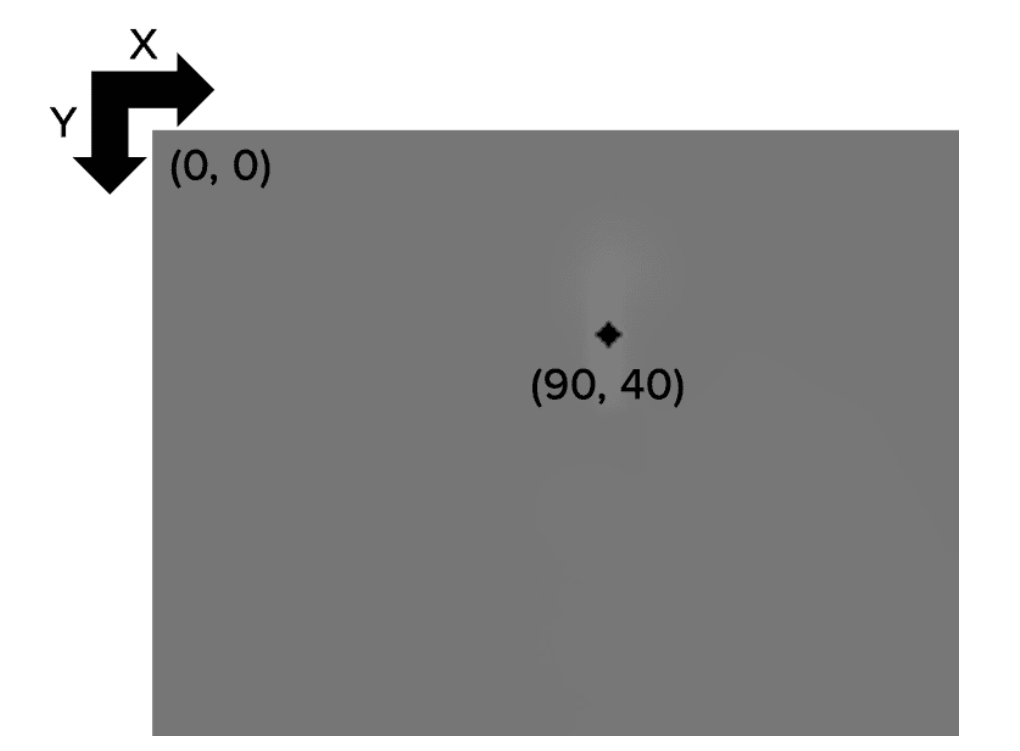# pixel coordinates
x = 90
y = 40

pixel_flame_gray16 = gray16_image [y, x]


# images are simply NumPy arrays -- with the origin (0, 0) located at
# the top-left of the image
(b, g, r) = image[0, 0]
print("Pixel at (0, 0) - Red: {}, Green: {}, Blue: {}".format(r, g, b))


## 读取文件

import argparse
import cv2
# construct the argument parser and parse the arguments
ap = argparse.ArgumentParser()
help="path to input image")
args = vars(ap.parse_args())

# load the image from disk via "cv2.imread" and then grab the spatial
# dimensions, including width, height, and number of channels
(h, w, c) = image.shape[:3]
# display the image width, height, and number of channels to our
# terminal
print("width: {} pixels".format(w))
print("height: {}  pixels".format(h))
print("channels: {}".format(c))

# show the image and wait for a keypress
cv2.imshow("Image", image)
cv2.waitKey(0)
# save the image back to disk (OpenCV handles converting image
# filetypes automatically)
cv2.imwrite("newimage.jpg", image)



## 裁剪操作

OpenCV将图像表示为NumPy数组，高度在前(行编号#)，宽度在后(列编号#)。

cropped = image[startY:endY, startX:endX]

import cv2

# load the input image and display it to our screen
cv2.imshow("Original", image)
# cropping an image with OpenCV is accomplished via simple NumPy
# array slices in startY:endY, startX:endX order -- here we are
# cropping the face from the image (these coordinates were
# determined using photo editing software such as Photoshop,
# GIMP, Paint, etc.)
face = image[85:250, 85:220]
cv2.imshow("Face", face)
cv2.waitKey(0)


## 算数操作

• OpenCV 执行剪切，并确保像素值永远不会超出范围[0,255]。
• NumPy 执行模数运算和循环。例如265表示为9
import numpy as np
import cv2

# images are NumPy arrays stored as unsigned 8-bit integers (unit8)
# with values in the range [0, 255]; when using the add/subtract
# functions in OpenCV, these values will be *clipped* to this range,
# even if they fall outside the range [0, 255] after applying the
# operation

subtracted = cv2.subtract(np.uint8(), np.uint8())
print("min of 0: {}".format(subtracted))
>>> max of 255: []
>>> min of 0: []

# using NumPy arithmetic operations (rather than OpenCV operations)
# will result in a modulo ("wrap around") instead of being clipped
# to the range [0, 255]

subtracted = np.uint8() - np.uint8()
print("wrap around: {}".format(subtracted))
>>> wrap around: 
>>> wrap around: 


## 位运算AND, OR, XOR, and NOT

opencv中处理逻辑运算使用命令bitwise_and, bitwise_or, bitwise_xor, bitwise_not.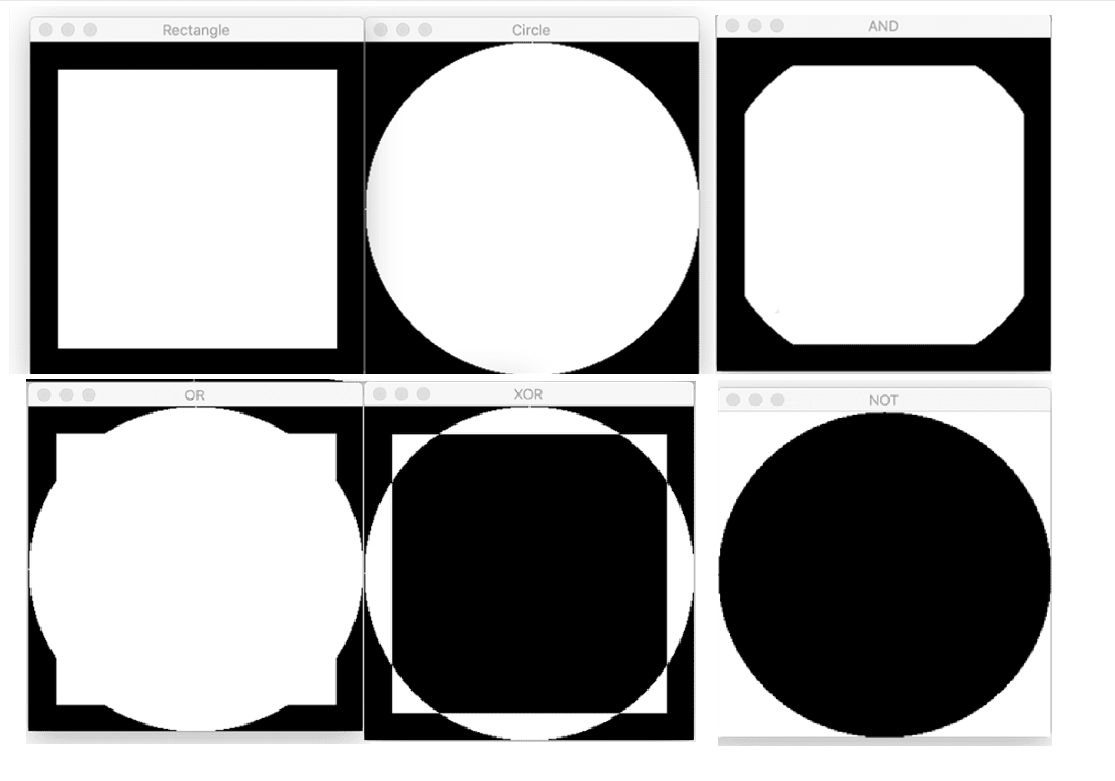import numpy as np
import cv2
# draw a rectangle
rectangle = np.zeros((300, 300), dtype="uint8")
cv2.rectangle(rectangle, (25, 25), (275, 275), 255, -1)
cv2.imshow("Rectangle", rectangle)
# draw a circle
circle = np.zeros((300, 300), dtype = "uint8")
cv2.circle(circle, (150, 150), 150, 255, -1)
cv2.imshow("Circle", circle)

# a bitwise 'AND' is only 'True' when both inputs have a value that
# is 'ON' -- in this case, the cv2.bitwise_and function examines
# every pixel in the rectangle and circle; if *BOTH* pixels have a
# value greater than zero then the pixel is turned 'ON' (i.e., 255)
# in the output image; otherwise, the output value is set to
# 'OFF' (i.e., 0)
bitwiseAnd = cv2.bitwise_and(rectangle, circle)
cv2.imshow("AND", bitwiseAnd)
cv2.waitKey(0)

# a bitwise 'OR' examines every pixel in the two inputs, and if
# *EITHER* pixel in the rectangle or circle is greater than 0,
# then the output pixel has a value of 255, otherwise it is 0
bitwiseOr = cv2.bitwise_or(rectangle, circle)
cv2.imshow("OR", bitwiseOr)
cv2.waitKey(0)

# the bitwise 'XOR' is identical to the 'OR' function, with one
# exception: the rectangle and circle are not allowed to *BOTH*
# have values greater than 0 (only one can be 0)
bitwiseXor = cv2.bitwise_xor(rectangle, circle)
cv2.imshow("XOR", bitwiseXor)
cv2.waitKey(0)

# finally, the bitwise 'NOT' inverts the values of the pixels;
# pixels with a value of 255 become 0, and pixels with a value of 0
# become 255
bitwiseNot = cv2.bitwise_not(circle)
cv2.imshow("NOT", bitwiseNot)
cv2.waitKey(0)


## 遮盖图像

import numpy as np
import cv2
cv2.imshow("Original", image)
# a mask is the same size as our image, but has only two pixel
# values, 0 and 255 -- pixels with a value of 0 (background) are
# ignored in the original image while mask pixels with a value of
# 255 (foreground) are allowed to be kept
cv2.rectangle(mask, (0, 90), (290, 450), 255, -1)
# apply our mask -- notice how only the person in the image is
# cropped out
cv2.waitKey(0)


## 缩放scaling(resize)

### cv2.resize

aspect_ratio = image_width / image_height

import numpy as np
import cv2

# 调整为150像素宽度
r = 150.0 / image.shape
dim = (150, int(image.shape * r))
# perform the actual resizing of the image
resized = cv2.resize(image, dim, interpolation=cv2.INTER_AREA)
cv2.imshow("Resized (Width)", resized)

# 调整为50像素高度
r = 50.0 / image.shape
dim = (int(image.shape * r), 50)
# perform the resizing
resized = cv2.resize(image, dim, interpolation=cv2.INTER_AREA)
cv2.imshow("Resized (Height)", resized)
cv2.waitKey(0)


### imutils.resize

import imutils
import cv2
# calculating the ratio each and every time we want to resize an
# image is a real pain, so let's use the imutils convenience
# function which will *automatically* maintain our aspect ratio
# for us
resized = imutils.resize(image, width=100)
cv2.imshow("Resized via imutils", resized)
cv2.waitKey(0)


### 插值方法

cv2.INTER_NEAREST 最近邻域插值
cv2.INTER_LINEAR 双线性插值
cv2.INTER_AREA 区域间插值

cv2.INTER_CUBIC based on a 4 x 4 pixel neighbor
cv2.INTER_LANCZOS4 based on an 8 x 8 pixel neighborhood

## 图像翻转cv2.flip

0表示垂直翻转（x轴），1表示水平翻转（y轴），-1表示两种操作都进行（180度）

import cv2
# flip the image horizontally
print("[INFO] flipping image horizontally...")
flipped = cv2.flip(image, 1)
cv2.imshow("Flipped Horizontally", flipped)

# flip the image vertically
flipped = cv2.flip(image, 0)
print("[INFO] flipping image vertically...")
cv2.imshow("Flipped Vertically", flipped)

# flip the image along both axes
flipped = cv2.flip(image, -1)
print("[INFO] flipping image horizontally and vertically...")
cv2.imshow("Flipped Horizontally & Vertically", flipped)
cv2.waitKey(0)


## 图像旋转

### cv2.rotate

python
import cv2
# grab the dimensions of the image and calculate the center of the
# image
(h, w) = image.shape[:2]
(cX, cY) = (w // 2, h // 2)
# rotate our image by 45 degrees around the center of the image
M = cv2.getRotationMatrix2D((cX, cY), 45, 1.0)
rotated = cv2.warpAffine(image, M, (w, h))
cv2.imshow("Rotated by 45 Degrees", rotated)
# rotate our image by -90 degrees around the image
M = cv2.getRotationMatrix2D((cX, cY), -90, 1.0)
rotated = cv2.warpAffine(image, M, (w, h))
cv2.imshow("Rotated by -90 Degrees", rotated)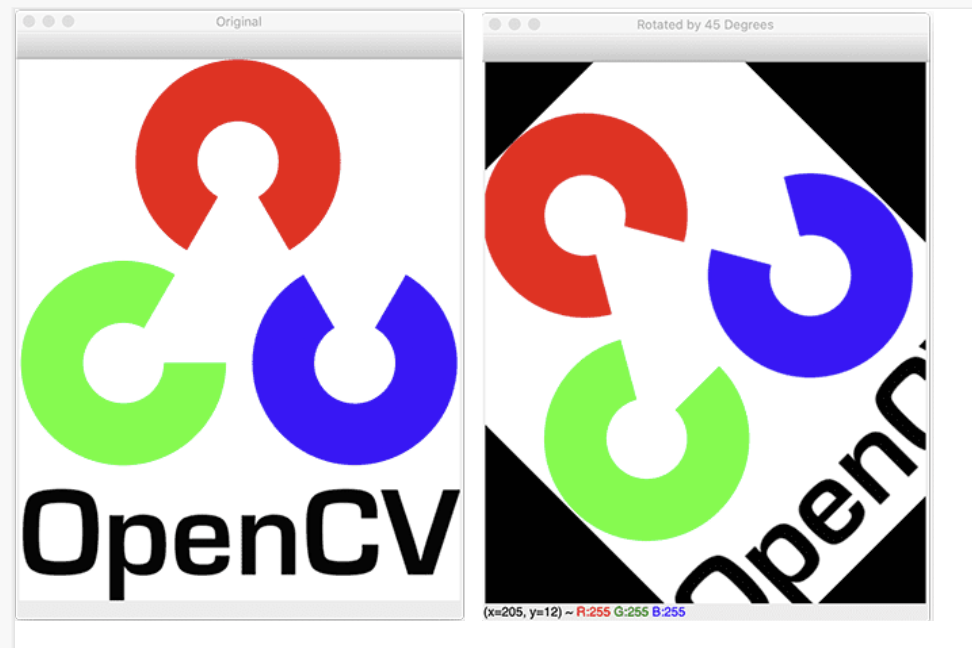### imutils.rotate和imutils.rotate_bound

imutils.convenience.rotate 为一个封装的方法，代码显得简化许多。

import cv2
import imutils

# use our imutils function to rotate an image 180 degrees
rotated = imutils.rotate(image, 180)
cv2.imshow("Rotated by 180 Degrees", rotated)


# rotate our image by 33 degrees counterclockwise, ensuring the
# entire rotated image still renders within the viewing area
rotated = imutils.rotate_bound(image, -33)
cv2.imshow("Rotated Without Cropping", rotated)
cv2.waitKey(0)


## 分离合并通道

import cv2
import imutils
# load the input image and grab each channel -- note how OpenCV
# represents images as NumPy arrays with channels in Blue, Green,
# Red ordering rather than Red, Green, Blue
(B, G, R) = cv2.split(image)
# show each channel individually
cv2.imshow("Red", R)
cv2.imshow("Green", G)
cv2.imshow("Blue", B)
cv2.waitKey(0)

# merge the image back together again
merged = cv2.merge([B, G, R])
cv2.imshow("Merged", merged)
cv2.waitKey(0)
cv2.destroyAllWindows()


## 画图功能

• cv2.line : 在图像上绘制一条直线，从指定的(x, y)坐标开始，结束于另一个(x, y)坐标
• cv2.circle : 在由中心(x, y)坐标和提供的半径指定的图像上绘制一个圆
• cv2.rectangle : 在左上角和右下角(x, y)坐标指定的图像上绘制矩形
还有其他命令
• cv2.ellipse : 绘制椭圆形
• cv2.polylines : 绘制由一组(x, y)坐标指定的多边形的轮廓
• cv2.fillPoly : 绘制一个多边形，但不是绘制轮廓，而是填充多边形
• cv2.arrowedLine : 绘制一个箭头，从起始(x, y)坐标指向结束(x, y)坐标
# import the necessary packages
import numpy as np
import cv2
# initialize our canvas as a 300x300 pixel image with 3 channels
# (Red, Green, and Blue) with a black background
canvas = np.zeros((300, 300, 3), dtype="uint8")

# draw a green line from the top-left corner of our canvas to the
# bottom-right
green = (0, 255, 0)
cv2.line(canvas, (0, 0), (300, 300), green)
cv2.imshow("Canvas", canvas)
cv2.waitKey(0)
# draw a 3 pixel thick red line from the top-right corner to the
# bottom-left
red = (0, 0, 255)
cv2.line(canvas, (300, 0), (0, 300), red, 3)
cv2.imshow("Canvas", canvas)
cv2.waitKey(0)

# draw a green 50x50 pixel square, starting at 10x10 and ending at 60x60
cv2.rectangle(canvas, (10, 10), (60, 60), green)
cv2.imshow("Canvas", canvas)
cv2.waitKey(0)
# draw another rectangle, this one red with 5 pixel thickness
cv2.rectangle(canvas, (50, 200), (200, 225), red, 5)
cv2.imshow("Canvas", canvas)
cv2.waitKey(0)
# draw a final rectangle (blue and filled in )
blue = (255, 0, 0)
cv2.rectangle(canvas, (200, 50), (225, 125), blue, -1) #-1表示全部填充
cv2.imshow("Canvas", canvas)
cv2.waitKey(0)


# re-initialize our canvas once again
canvas = np.zeros((300, 300, 3), dtype="uint8")
# let's draw 25 random circles
for i in range(0, 25):
# randomly generate a radius size between 5 and 200, generate a
# random color, and then pick a random point on our canvas where
# the circle will be drawn
color = np.random.randint(0, high=256, size=(3,)).tolist()
pt = np.random.randint(0, high=300, size=(2,))
# draw our random circle on the canvas
# display our masterpiece to our screen
cv2.imshow("Canvas", canvas)
cv2.waitKey(0)


## 图像平移

M = np.float32([
[1, 0, shiftX],
[0, 1, shiftY]
])

import cv2
# shift the image 25 pixels to the right and 50 pixels down
M = np.float32([[1, 0, 25], [0, 1, 50]])
shifted = cv2.warpAffine(image, M, (image.shape, image.shape))
cv2.imshow("Shifted Down and Right", shifted)


# use the imutils helper function to translate the image 100 pixels
# down in a single function call
shifted = imutils.translate(image, 0, 100)
cv2.imshow("Shifted Down", shifted)
cv2.waitKey(0)
`
]]>
<![CDATA[2023年Flag]]> https://blog.laffitto.xyz/post/2023-nian-flag/ 2023-01-30T13:13:36.000Z 用OKR方式来指定一下新一年目标

]]>

## OKR

OKR（Objectives and Key Results） 目标与关键成果法，这其中：

• O是目标，回答的是「我想要完成什么？」，是某个时间段内的目标和任务。目标的前提由动机可行性组成。
• KR是一系列可衡量的关键结果，回答的是「我如何知道自己是否达成了目标？」。这其中可以使用限制词将具体的事转变为结果。

## 自己的OKR规划

### O1 职业发展 精进技术，找新工作

#### KR1 继续完善专业相关知识

##### A3 学习精进深度学习技术

pytorch的代码技术水平一般，需要多读读别人的相关与源码，同时技术革新较快，需要大致了解一下其他技术的更迭项目以保证不落后。包括但不仅限于分类任务，分割任务，检测任务等等。同时OpenCV也希望好好学习一下。

## 总结

]]>
<![CDATA[2022年度总结]]> https://blog.laffitto.xyz/post/2022-nian-du-zong-jie/ 2022-12-25T08:35:35.000Z 又是一年过去了，再见2022~

]]>

## 精神世界

]]>
<![CDATA[生命之轻]]> https://blog.laffitto.xyz/post/sheng-ming-zhi-qing/ 2022-11-27T08:00:02.000Z 有些只有发生在身边的事情才会感觉到真实

]]>

“不知道你还记得妞妞嘛，她妈妈死了。”

“这么突然的嘛”，我先是一愣，“是住在5楼的那户嘛？”

“是的，据说是子宫癌，开刀直接在没下的来手术台”。妈妈叹了口气，说道。

“还有那个谁，不知道你记得不，XXX，他昨天也死了。也不知道什么原因，可能是高血压糖尿病并发症发作”，姥姥补充道，“他们家人都不敢告诉他奶奶，怕他奶奶承受不住。”

“他生下来时候就一身的病，妈妈当时生他时候就去世了，他爷爷为了给他看病，卖了好多东西，为了保住这个小孙子。他爷爷之前可有钱了，为了小孙子也是散尽了家财，能长这么大都是拿钱换来的，可惜现在也死了。之前就看他那个腿，都是紫的，问他他自己也明白，说治不好的，自己倒是看的很开，总是笑嘻嘻的”。

“他多大了呀？”

“大概也就40岁不到吧，可怜了他还有个小儿子，看着白白净净怪可爱的。”说到这里我看到明显姥姥的眼眶中有泪水在打转。

“哎，至少他的小儿子还有爱他的妈妈和爷爷”，我安慰道，“而且他能活这么大，他家人应该也很开心了”。

]]>
<![CDATA[中秋轶事]]> https://blog.laffitto.xyz/post/zhong-qiu-yi-shi/ 2022-09-12T09:34:27.000Z 回家过了个中秋节

]]>

## 中秋礼物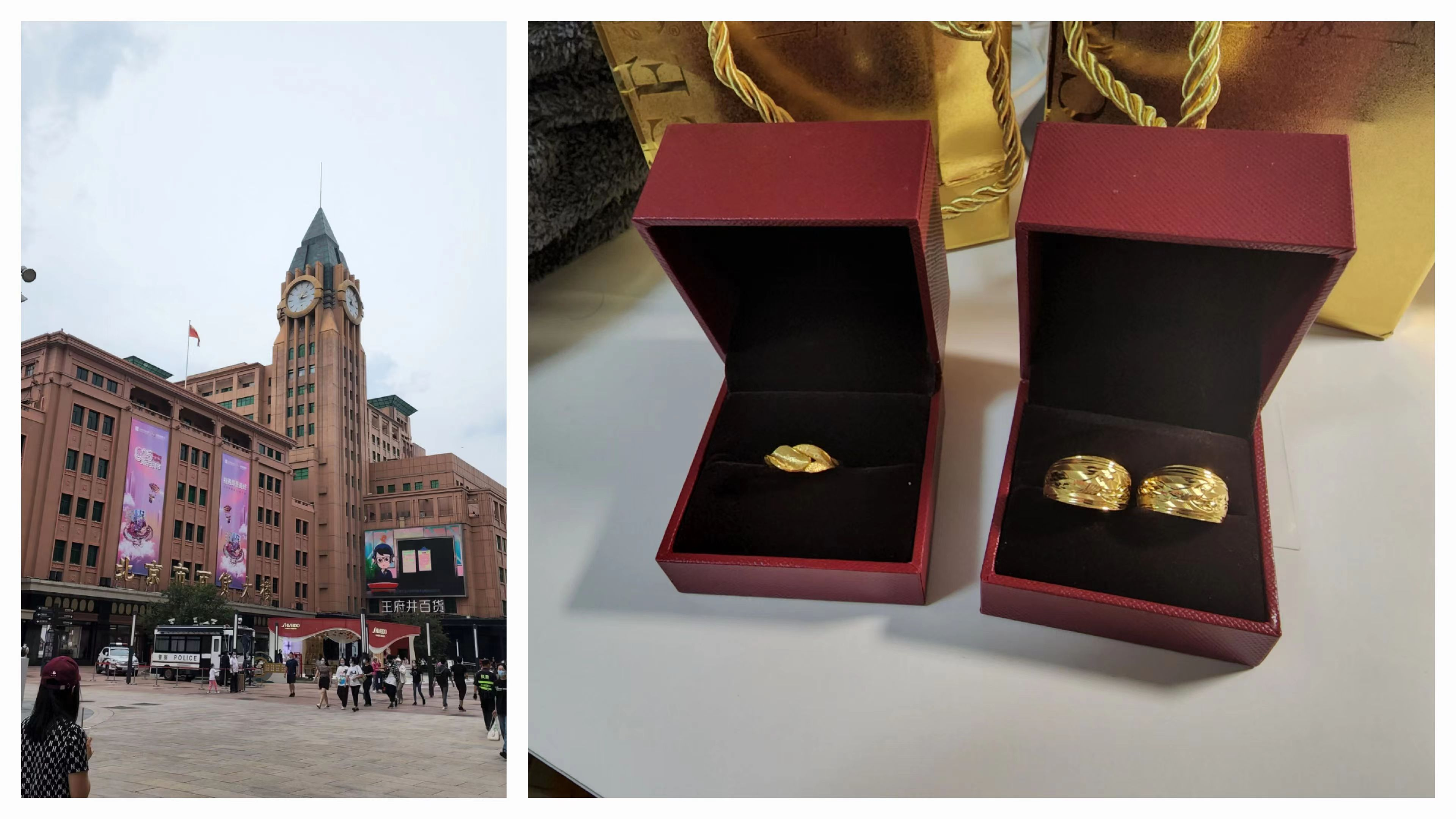## 回家旅途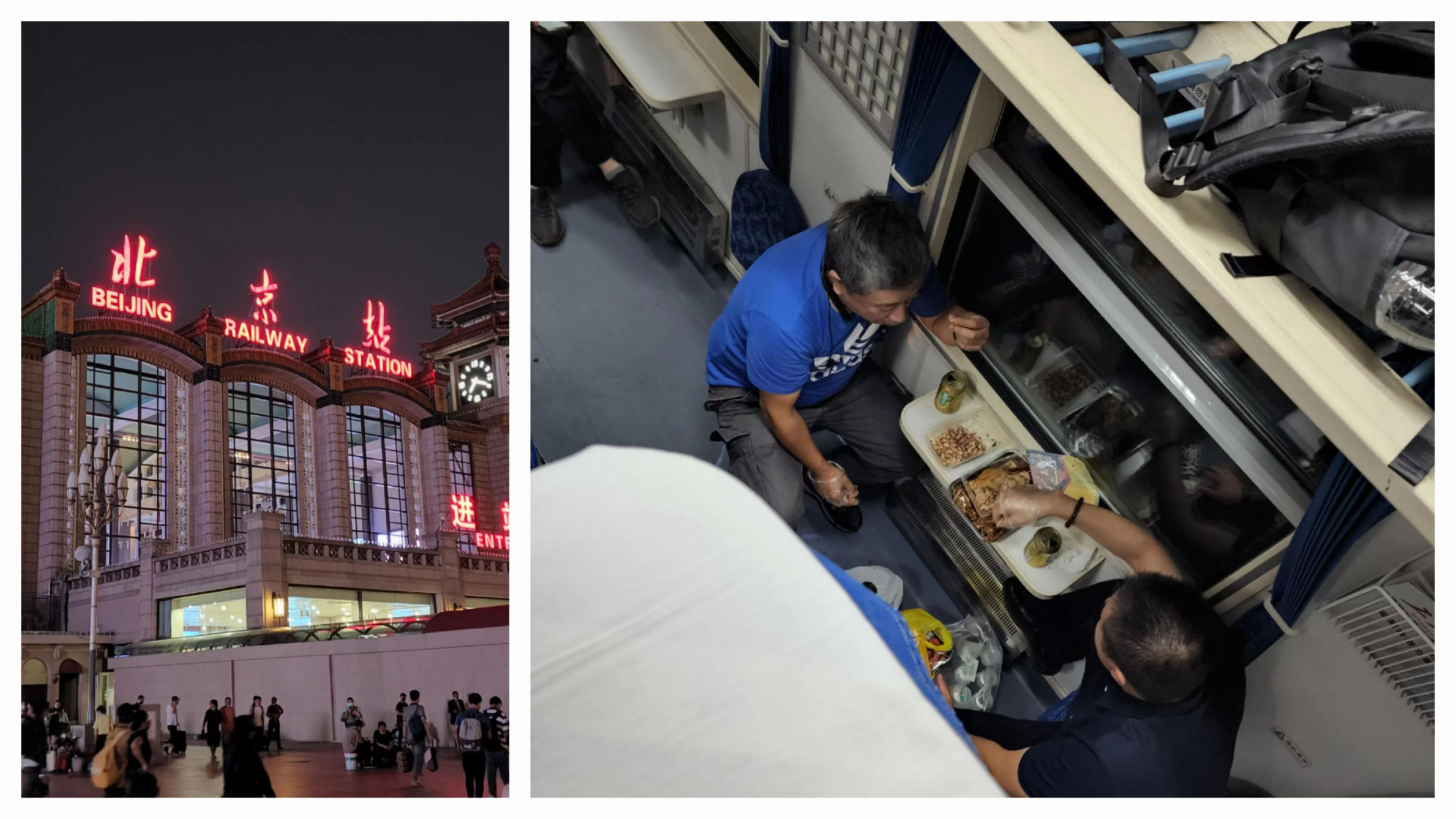## 中秋团圆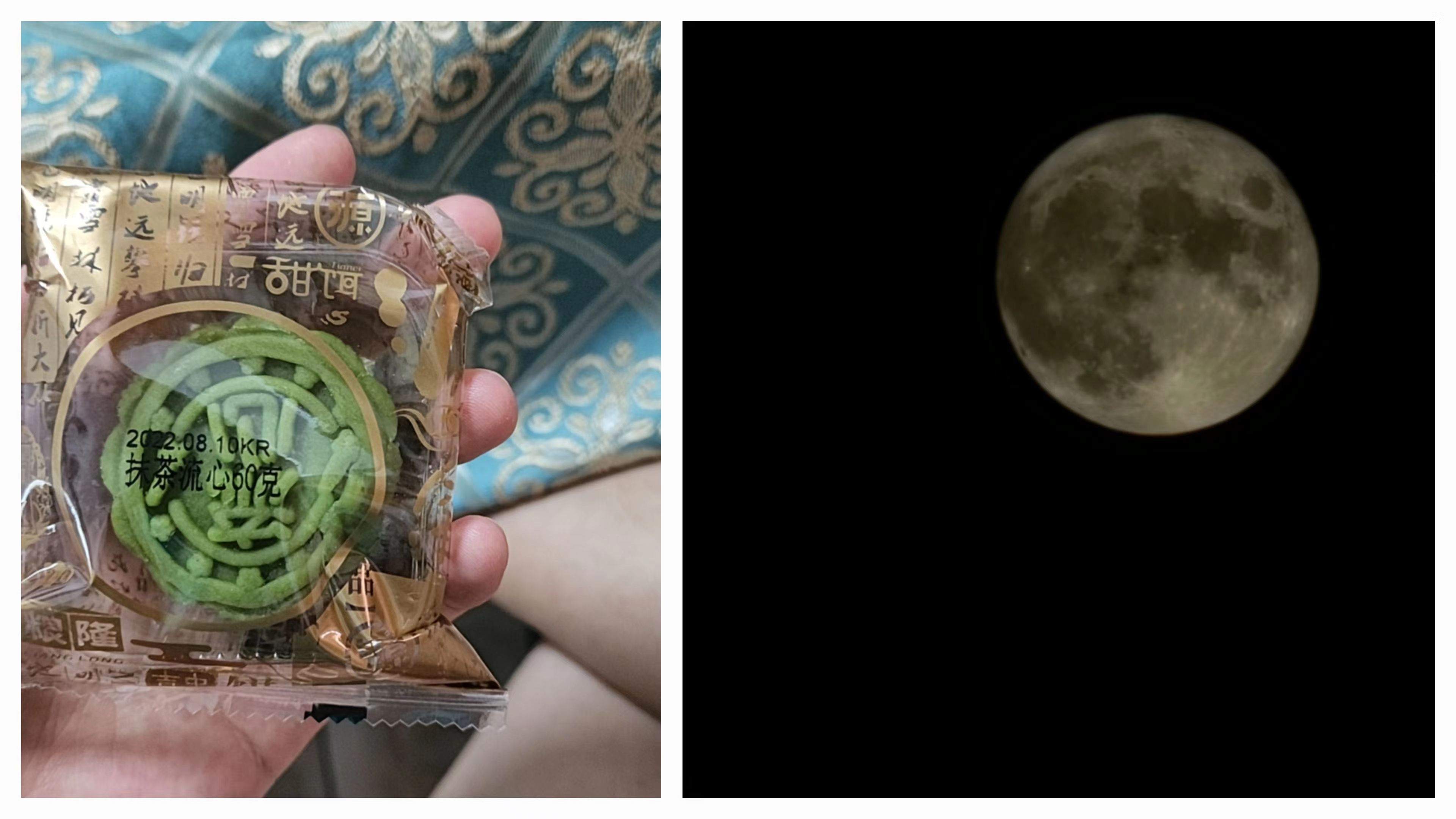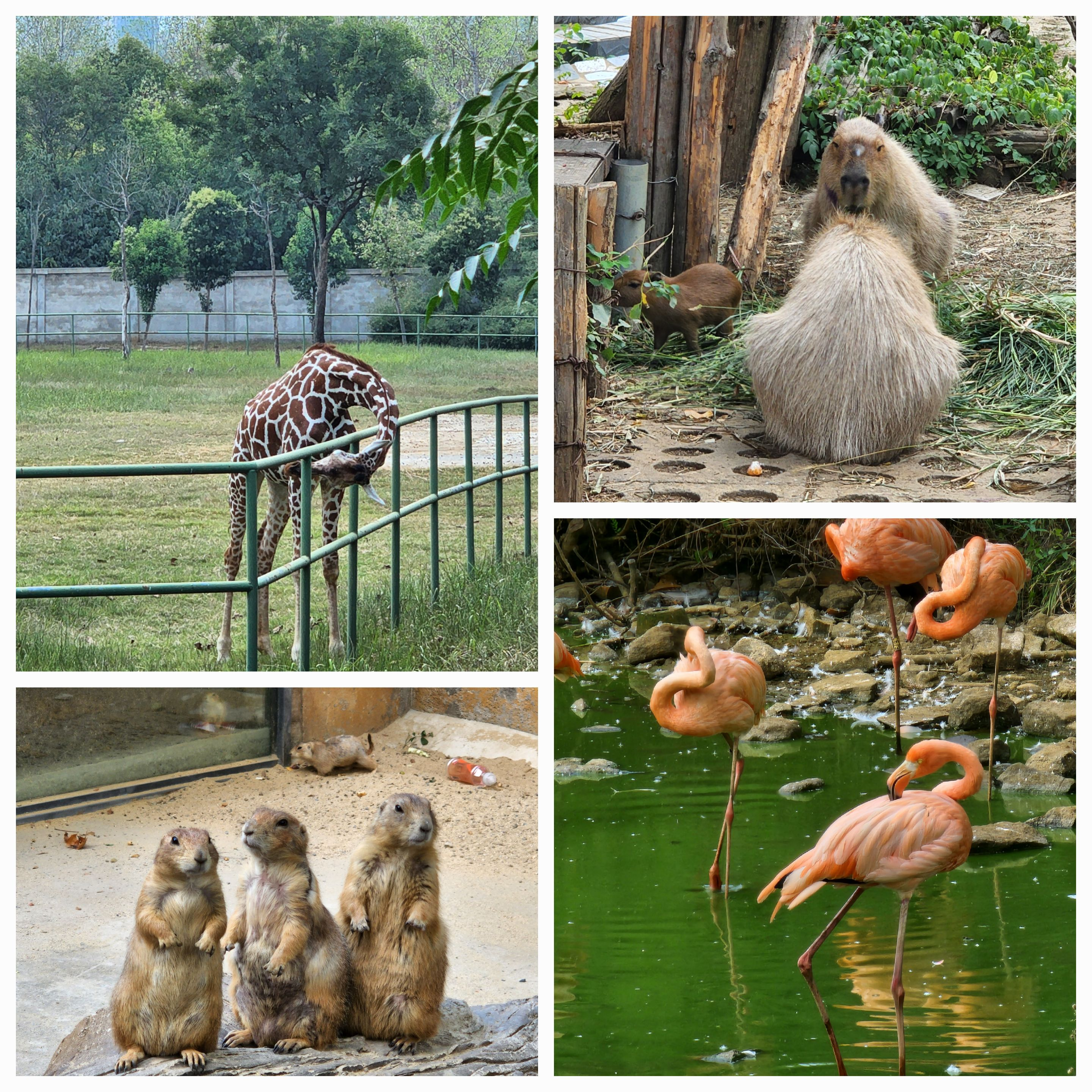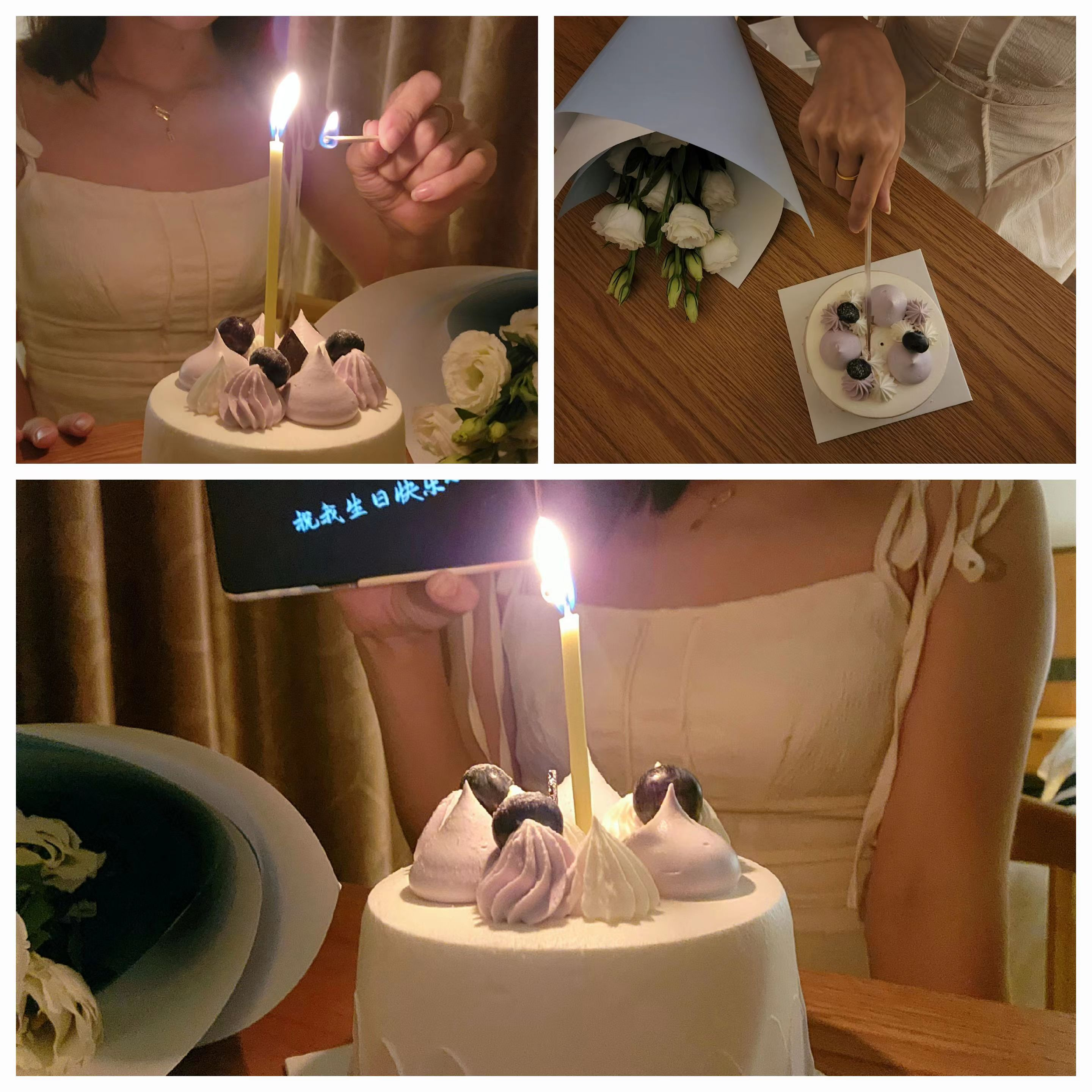## 返程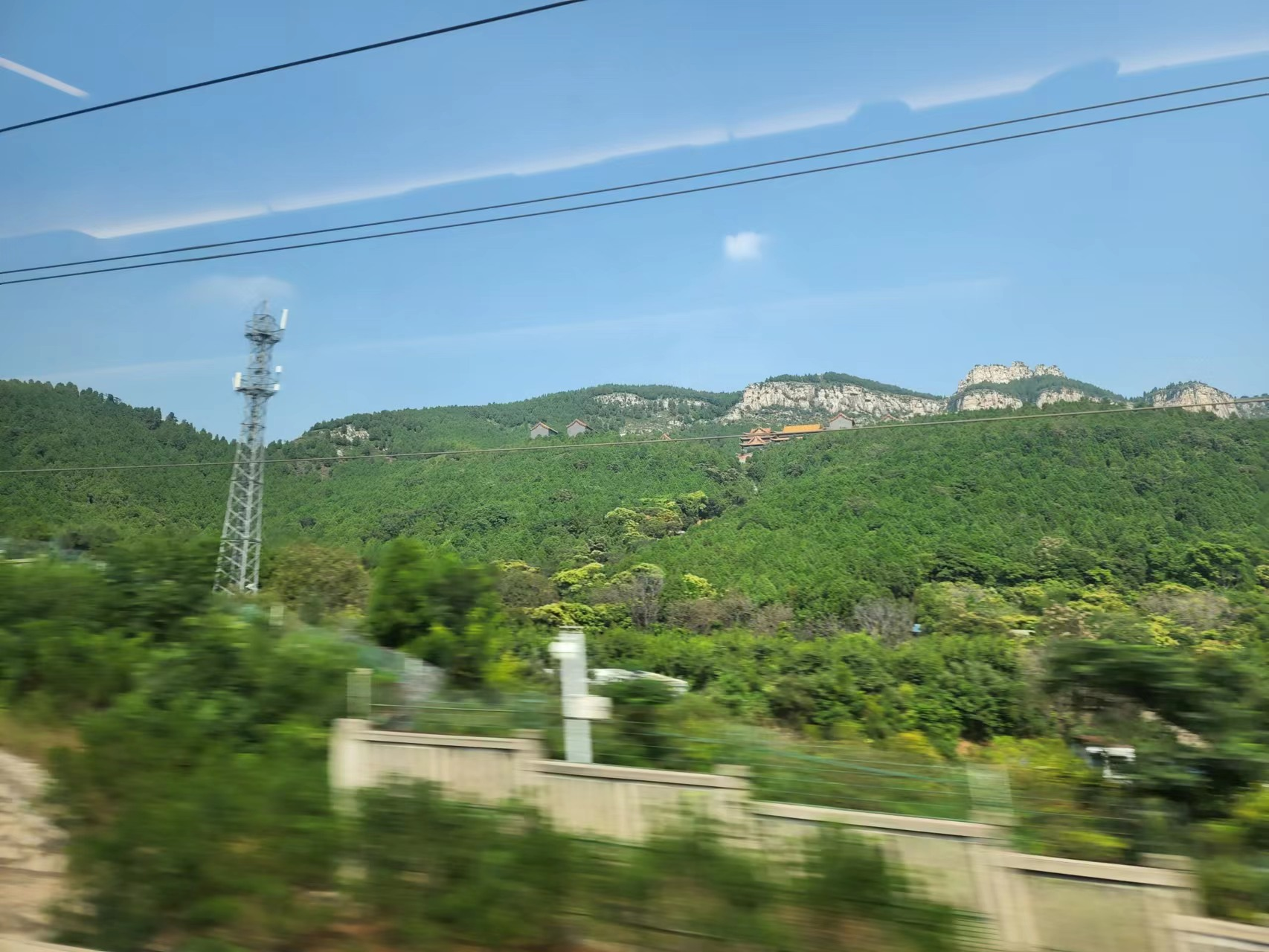2022年9月12日

]]>
<![CDATA[詹詹碎言 #5]]> https://blog.laffitto.xyz/post/zhan-zhan-sui-yan-5/ 2022-08-14T07:51:36.000Z 碎碎念

]]>

## 播客

]]>
<![CDATA[MRI简记]]> https://blog.laffitto.xyz/post/mri-jian-ji/ 2022-07-16T12:06:26.000Z 一些MRI常用序列

]]>

## 术语描述

### 强度/intensity

• high signal intensity = white
• intermediate signal intensity = grey
• low signal intensity = black

• hyperintense = brighter than the thing we are comparing it to
• isointense = same brightness as the thing we are comparing it to
• hypointense = darker than the thing we are comparing it to

tips： CT中描述强度使用density，避免混淆使用！

## MRI序列

MRI仅能探测横向宏观磁化矢量。而氢质子进入高能状态后，可以产生此类矢量。

### T1加权/T1 weighted

• 脂肪：白色 high signal intensity

• 肌肉：灰色 intermediate signal intensity

• 流体（尿液，脑脊液）：深色 low signal intensity

• 流动的血液：深色 low signal intensity

• 骨：深色

• 空气：深色

• 脑：

• 灰质：灰色

• 白质：明亮（与灰质相比高信号）hyperintense compared to grey matter (white-ish)

T1 最适合用于评估解剖结构，因为图像在宏观上类似于组织。

### T2加权/T2 weighted

• 脂肪：白色 high signal intensity
• 肌肉：灰色 intermediate signal intensity
• 流体（尿液，脑脊液）：白色 high signal intensity
• 流动的血液：深色
• 骨：深色
• 空气：深色
• 脑：
• 灰质：灰色
• 白质：灰暗（与灰质相比低信号）hypointense compared to grey matter (dark-ish)

#### Short Tau Inversion Recovery (STIR)

STIR 不能在注射钆后使用，因为钆的 T1 在相同的脂肪范围内，最终来自它的信号会减弱。

• 脂肪：深色
• 肌肉：比脂肪更黑
• 流体：非常明亮
• 骨：深色
• 空气：深色
• 脑：
• 灰质：灰色
• 白质：比灰质更暗

• 脂肪：白色
• 肌肉：灰色
• 流体：白色
• 骨：深色
• 空气：深色
• 透明软骨：灰色
• 纤维软骨：深色

### 扩散加权序列

• 液体（如尿液、脑脊液）：不限制扩散
• 软组织（肌肉、实体器官、脑）：中间扩散
• 脂肪：由于缺水，信号微弱

#### 传统DWI/diffusion weighted image（DWI）

DWI 专门检测水分子中质子的运动。它代表了实际扩散值和 T2 信号的组合。

• 脂肪：由于缺水，信号微弱
• 肌肉：灰色
• 流体（CFS）：深色
• 脑：
• 灰质：灰色
• 白质：灰暗（与灰质相比略低）

• CSF：高信号（白色）
• 脂肪：由于缺水，信号微弱
• 其他软组织：中等信号强度（灰色）
• 灰质：中间信号强度（灰色）
• 白质：与灰质相比略高

## 参考文章

]]>
<![CDATA[青岛之旅]]> https://blog.laffitto.xyz/post/qing-dao-zhi-lu/ 2022-06-25T12:08:17.000Z 大海真的好舒服啊~

]]>

## 基本准备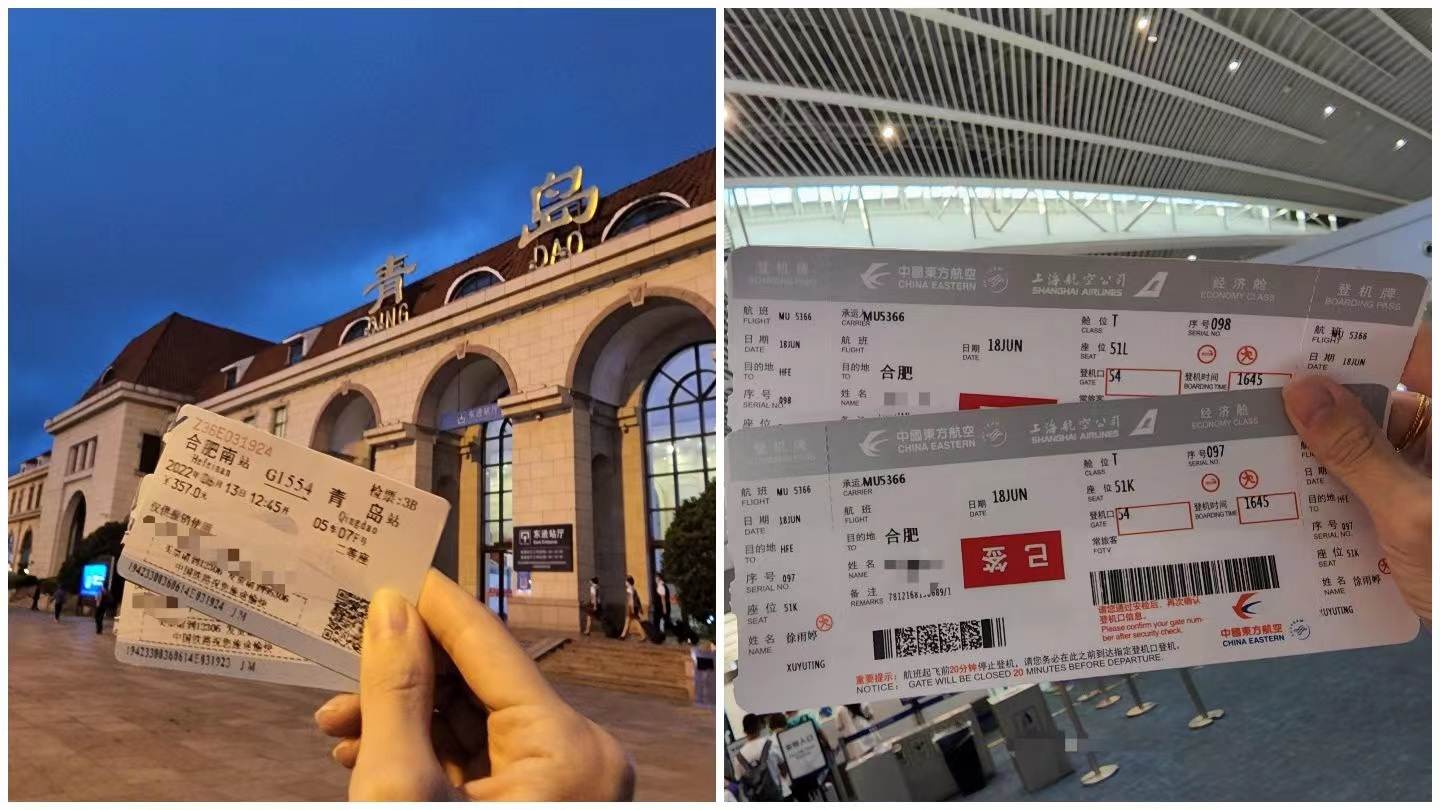## 沿途景色

### 青岛海昌极地海洋公园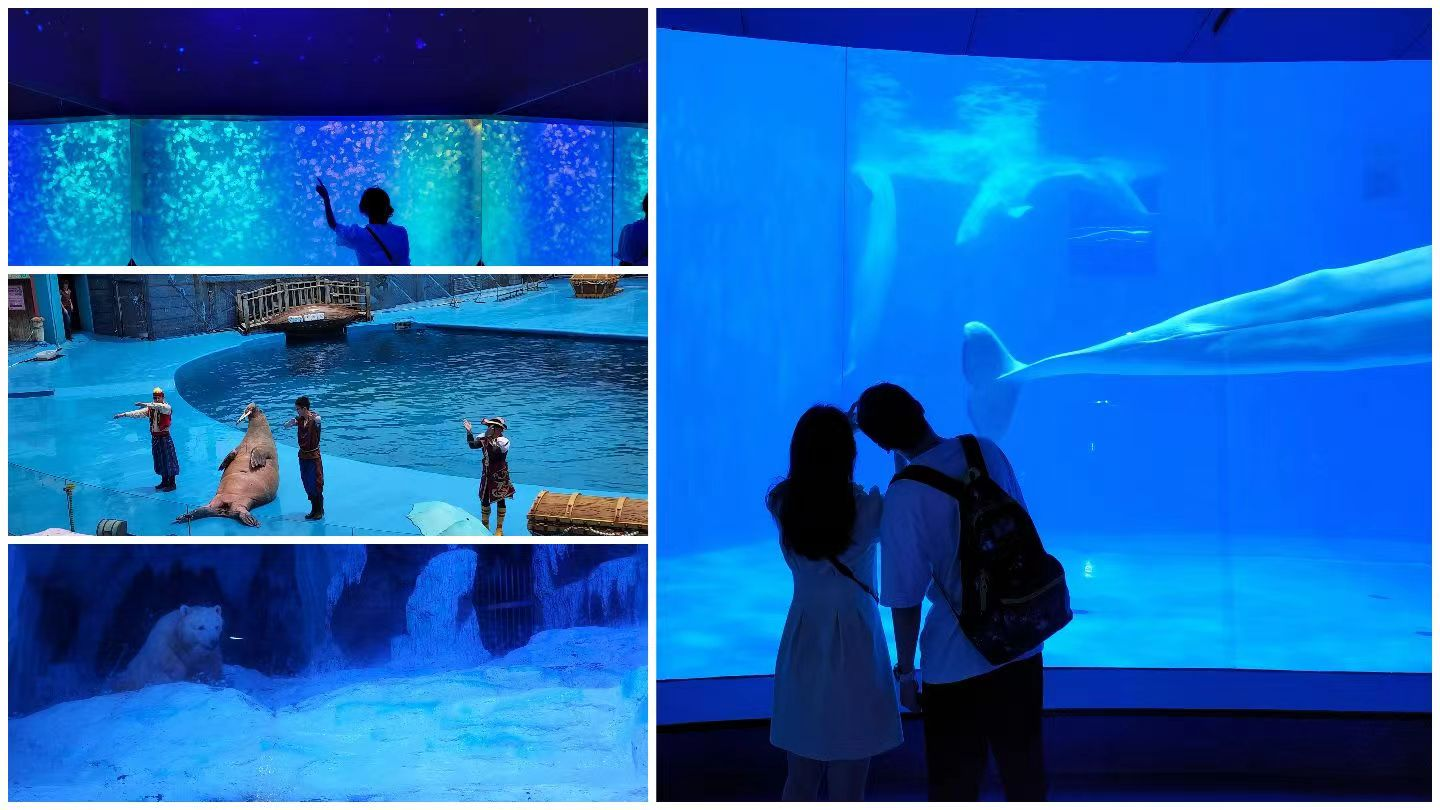### 青岛啤酒博物馆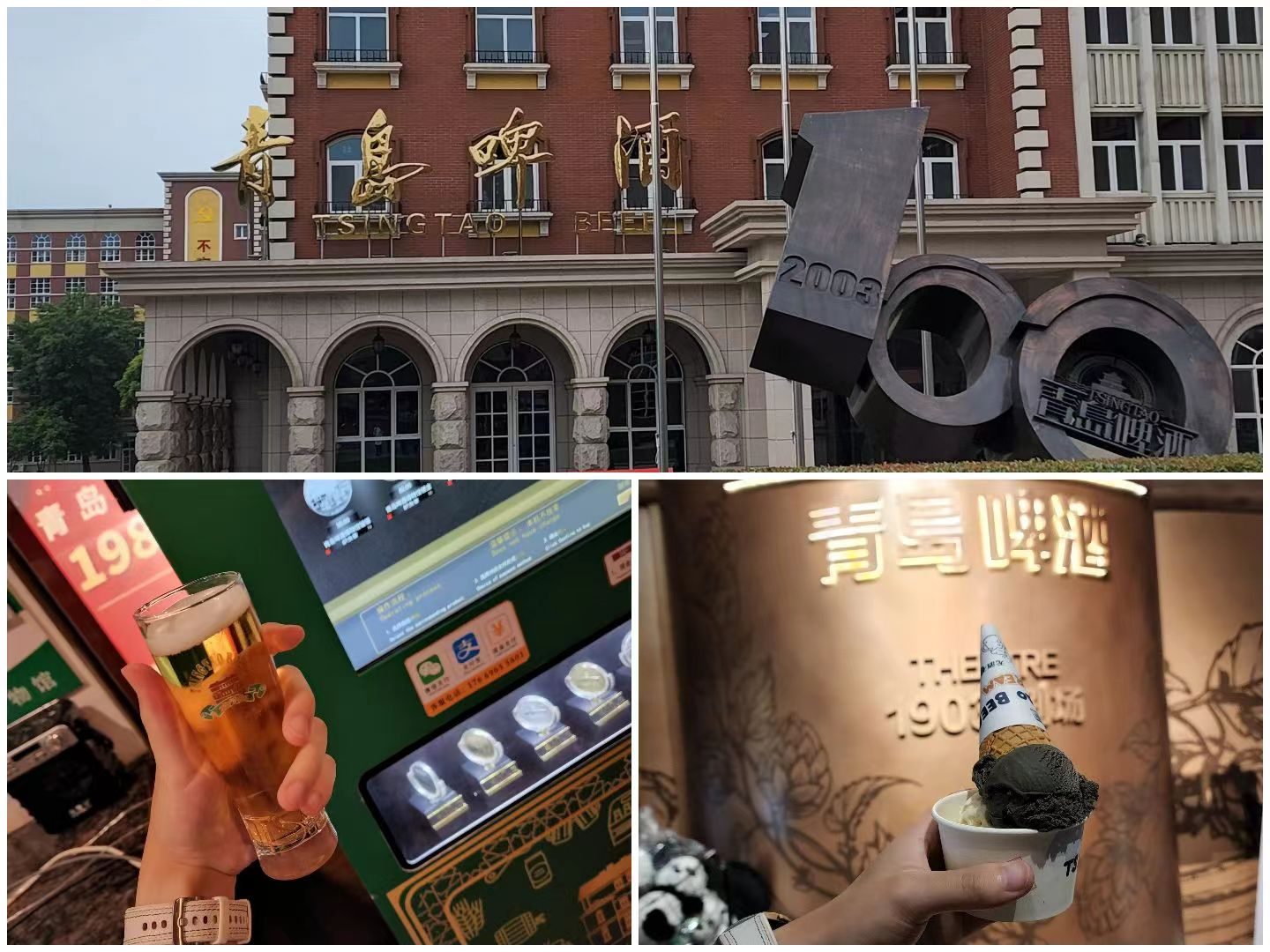### 崂山风景区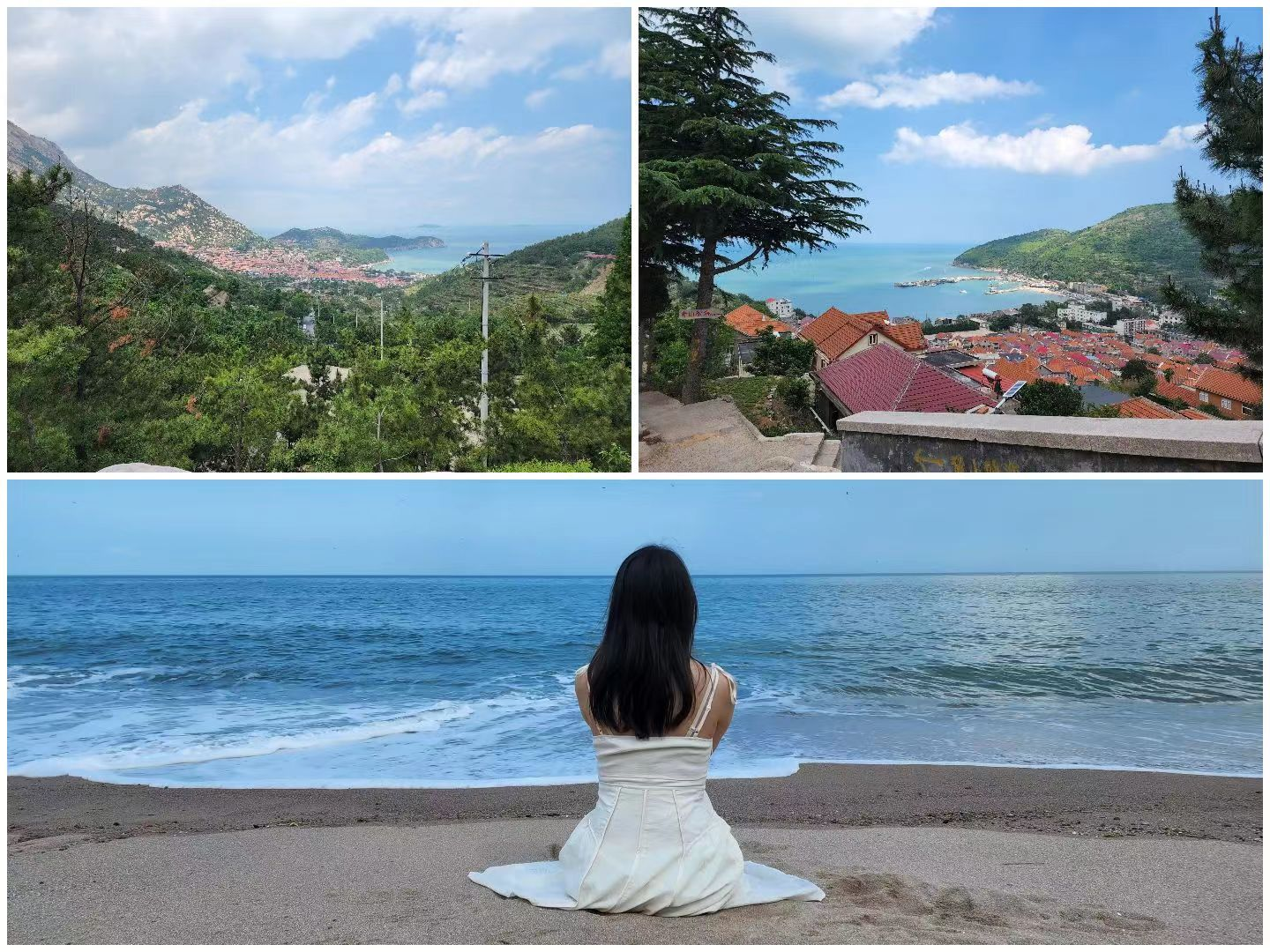### 五四广场灯光秀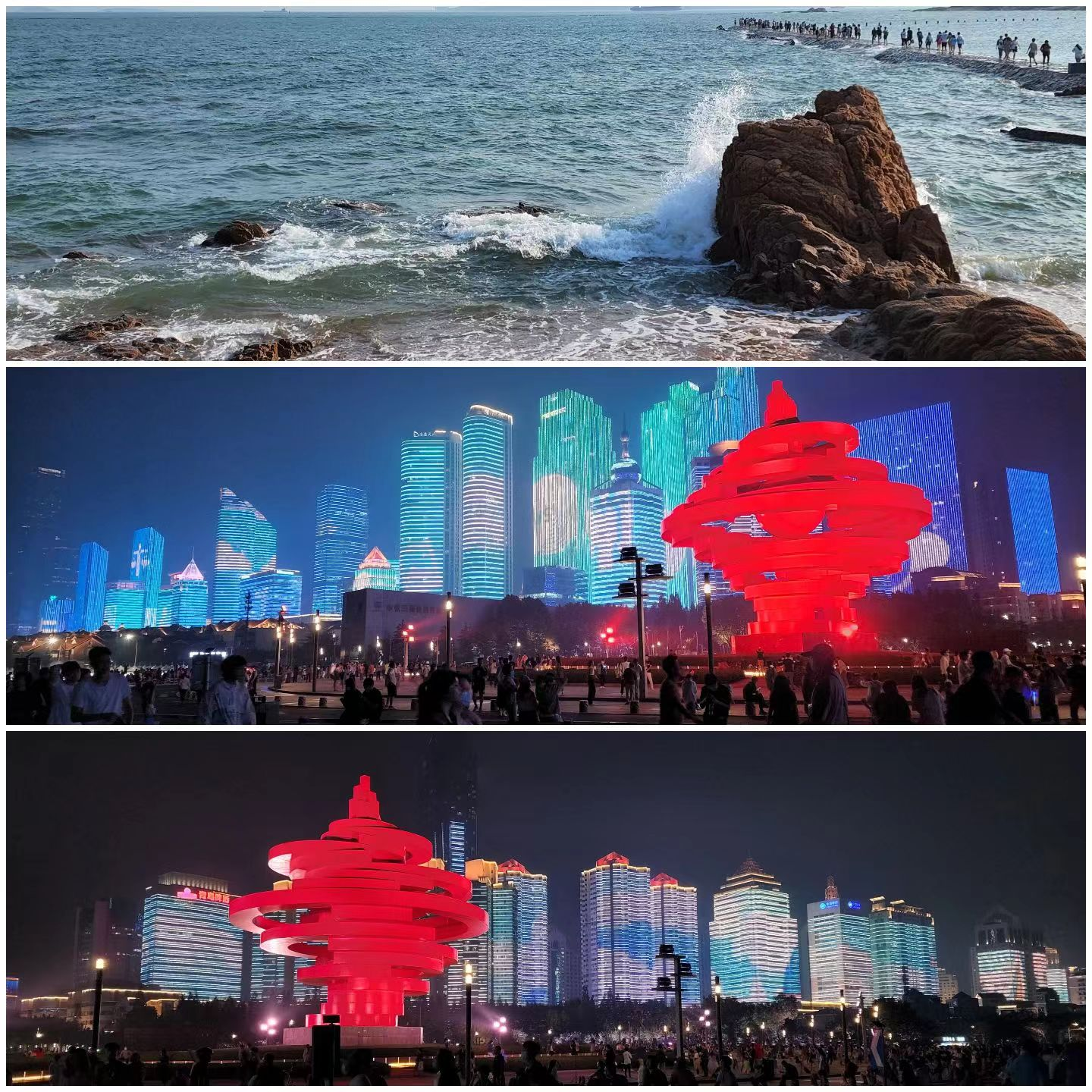## 城市印象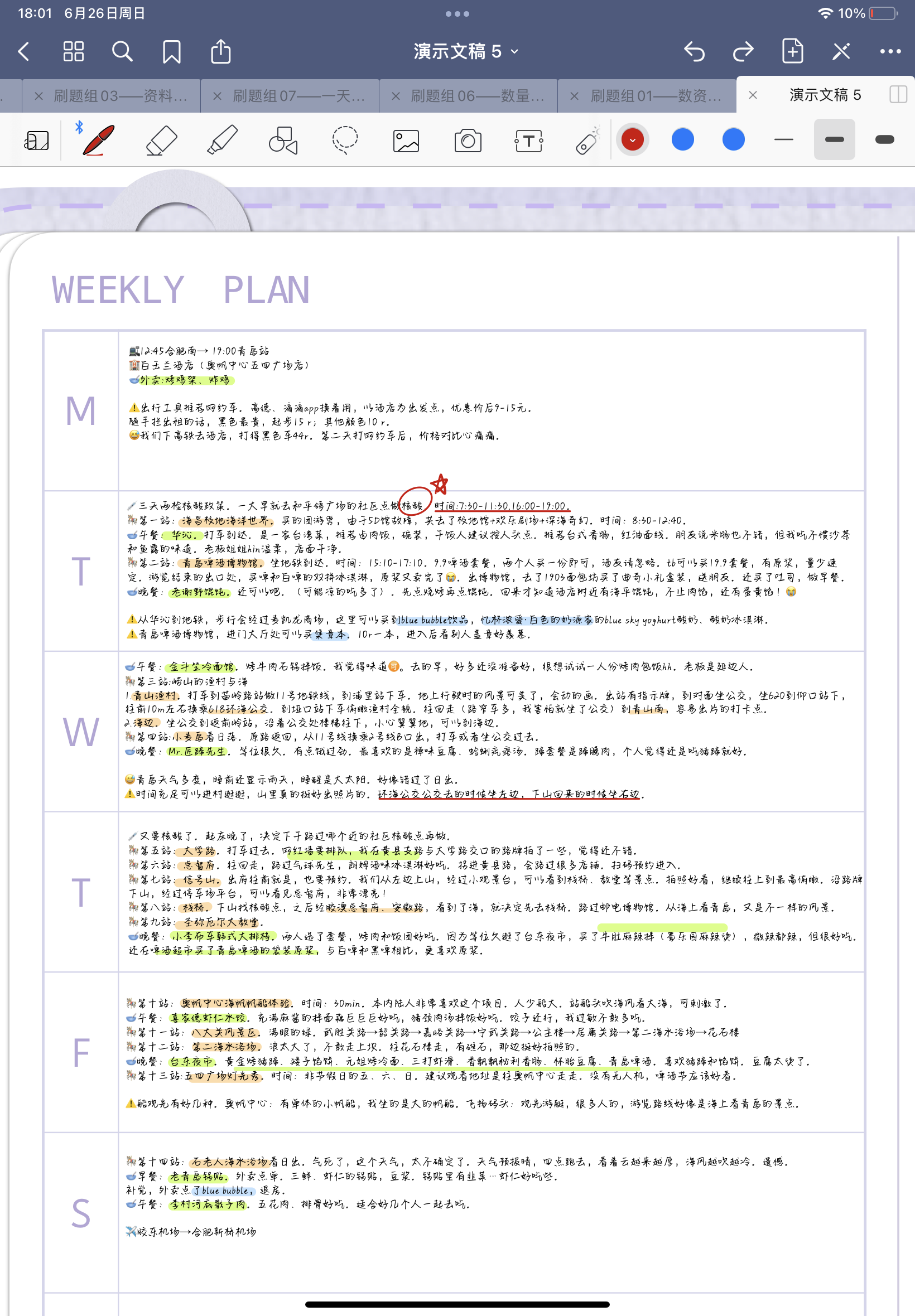]]>